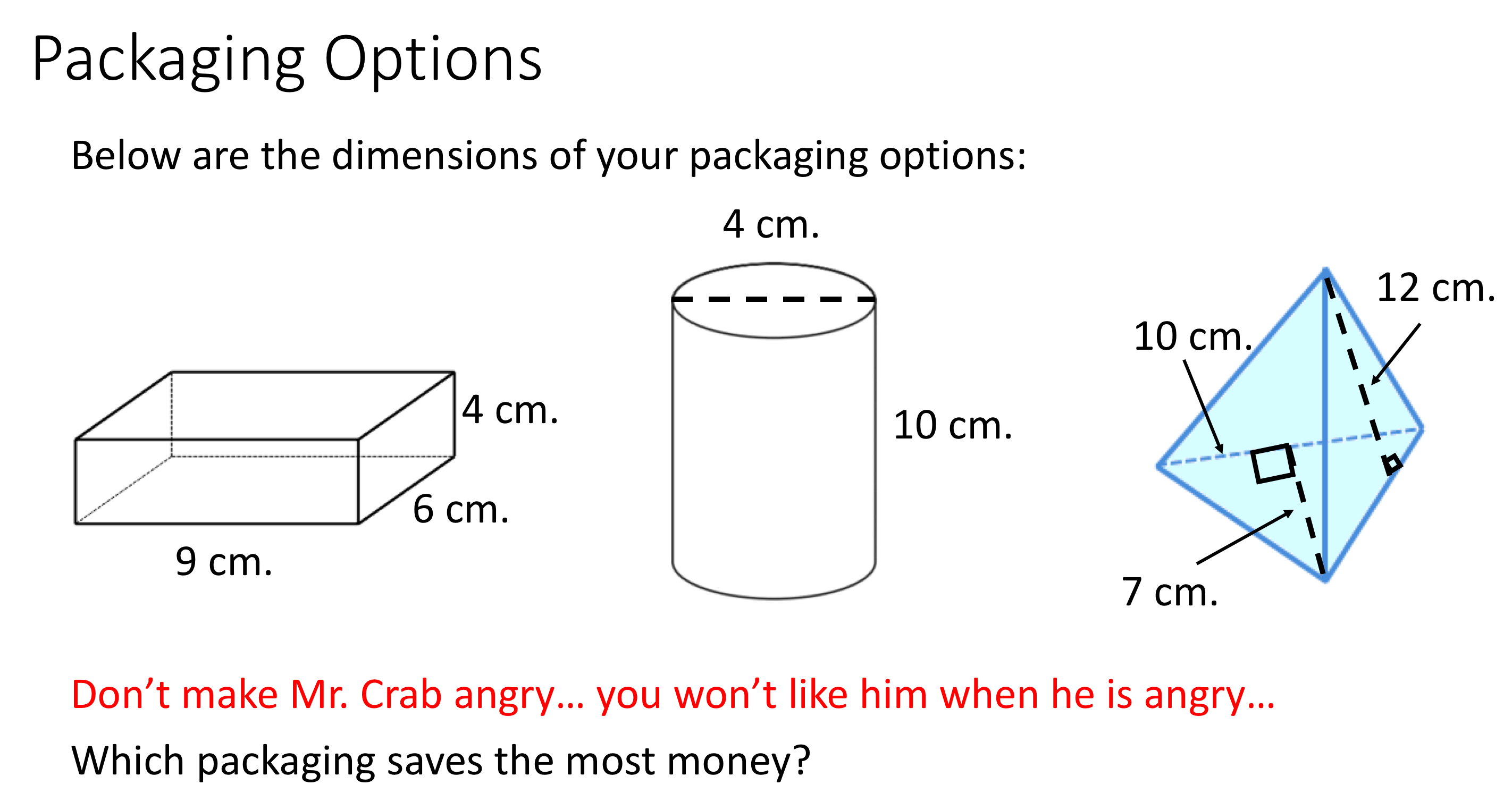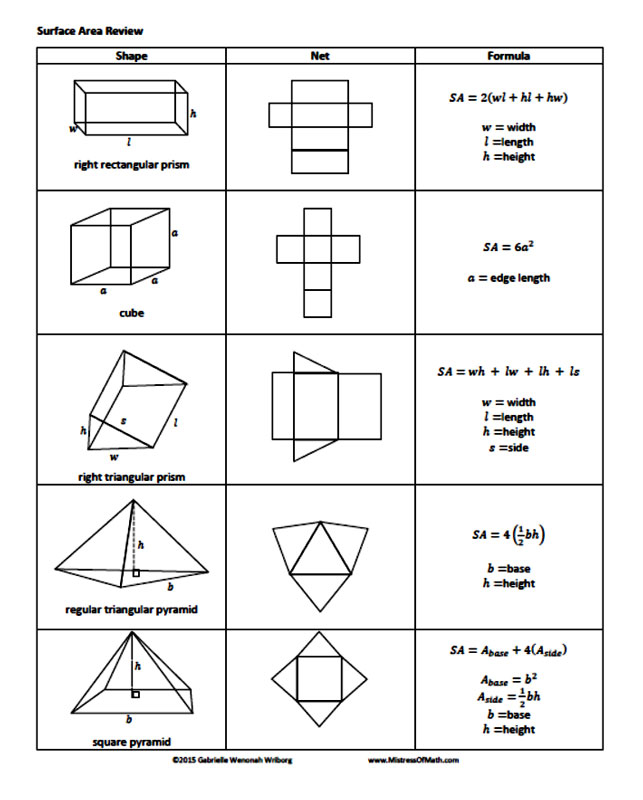Surface Area of Prisms, Pyramids, and Cylinders
starstarstarstarstarstarstarstarstarstar
by Felix Gabathuler
| 4 QuestionsTo solve for surface area, which is the the sum of the area of the faces of an object, an effective way to calculate this sum is to examine the object's net. Below you can find a list of common 3D shapes and their accompanying net.# Cylinder1
1
For the question above, what is the surface area of the prism?
2
1
For the question above, what is the surface area of the cylinder? Leave your answer in terms of pi.
3
1
For the question above, what is the surface area of the pyramid?
4
1
Which packaging option costs the least amount>
Cylindrical Option
Prism Option
Pyramid Option